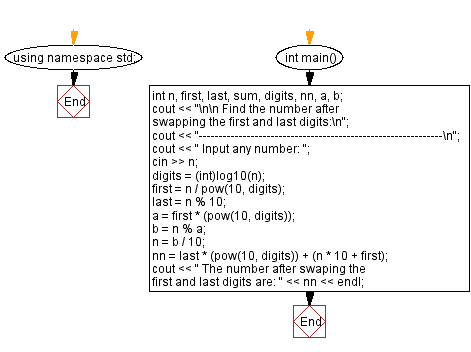﻿ C++ Exercises: Swap first and last digits of any number - w3resource

# C++ Exercises: Swap first and last digits of any number

## C++ Basic: Exercise-61 with Solution

Write a C++ program to swap the first and last digits of any number.

Sample Solution:

C++ Code :

``````#include <iostream>
#include <math.h>
using namespace std;

int main()
{
int n, first, last, sum, digits, nn, a, b;
cout << "\n\n Find the number after swapping the first and last digits:\n";
cout << "-------------------------------------------------------------\n";
cout << " Input any number: ";
cin >> n;
digits = (int)log10(n);
first = n / pow(10, digits);
last = n % 10;
a = first * (pow(10, digits));
b = n % a;
n = b / 10;
nn = last * (pow(10, digits)) + (n * 10 + first);
cout << " The number after swaping the first and last digits are: " << nn << endl;
}
``````

Sample Output:

```Find the number after swapping the first and last digits:
-------------------------------------------------------------
Input any number: 12345
The number after swaping the first and last digits are: 52341 ```

Flowchart:C++ Code Editor:

What is the difficulty level of this exercise?

﻿

## C++ Programming: Tips of the Day

Why is there no std::stou?

The most pat answer would be that the C library has no corresponding "strtou", and the C++11 string functions are all just thinly veiled wrappers around the C library functions: The std::sto* functions mirror strto*, and the std::to_string functions use sprintf.

Ref: https://bit.ly/3wtz2qA

We are closing our Disqus commenting system for some maintenanace issues. You may write to us at reach[at]yahoo[dot]com or visit us at Facebook